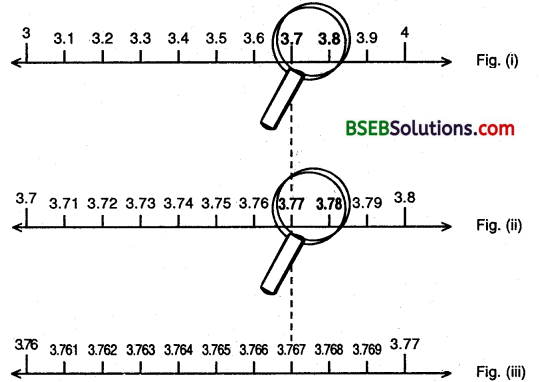# HSSlive: Plus One & Plus Two Notes & Solutions for Kerala State Board

## BSEB Class 9 Maths Chapter 1 Number Systems Ex 1.4 Textbook Solutions PDF: Download Bihar Board STD 9th Maths Chapter 1 Number Systems Ex 1.4 Book AnswersBSEB Class 9 Maths Chapter 1 Number Systems Ex 1.4 Textbook Solutions PDF: Download Bihar Board STD 9th Maths Chapter 1 Number Systems Ex 1.4 Book Answers

BSEB Class 9th Maths Chapter 1 Number Systems Ex 1.4 Textbooks Solutions and answers for students are now available in pdf format. Bihar Board Class 9th Maths Chapter 1 Number Systems Ex 1.4 Book answers and solutions are one of the most important study materials for any student. The Bihar Board Class 9th Maths Chapter 1 Number Systems Ex 1.4 books are published by the Bihar Board Publishers. These Bihar Board Class 9th Maths Chapter 1 Number Systems Ex 1.4 textbooks are prepared by a group of expert faculty members. Students can download these BSEB STD 9th Maths Chapter 1 Number Systems Ex 1.4 book solutions pdf online from this page.

## Bihar Board Class 9th Maths Chapter 1 Number Systems Ex 1.4 Books Solutions

 Board BSEB Materials Textbook Solutions/Guide Format DOC/PDF Class 9th Subject Maths Chapter 1 Number Systems Ex 1.4 Chapters All Provider Hsslive

## How to download Bihar Board Class 9th Maths Chapter 1 Number Systems Ex 1.4 Textbook Solutions Answers PDF Online?

2. Click on the Bihar Board Class 9th Maths Chapter 1 Number Systems Ex 1.4 Answers.
3. Look for your Bihar Board STD 9th Maths Chapter 1 Number Systems Ex 1.4 Textbooks PDF.
4. Now download or read the Bihar Board Class 9th Maths Chapter 1 Number Systems Ex 1.4 Textbook Solutions for PDF Free.

## BSEB Class 9th Maths Chapter 1 Number Systems Ex 1.4 Textbooks Solutions with Answer PDF Download

Find below the list of all BSEB Class 9th Maths Chapter 1 Number Systems Ex 1.4 Textbook Solutions for PDF’s for you to download and prepare for the upcoming exams:

## BSEB Bihar Board Class 9th Maths Solutions Chapter 1 Number Systems Ex 1.4

Question 1.
Visualise 3.765 on the number line, using successive magnification.
Solution:
We know that 3.765 lies between 3 and 4, that is, in the interval [3, 4] to have a rough idea where it is. Now, we divide the interval [3, 4] into 10 equal parts and look at [3.7, 3.8] through a magnifying glass and realize that 3.765 lies between 3.7 and 3.8 (see figure (i). Now, we imagine that each of the new intervals [3.1, 3.2], [3.2, 3.3], …, [3.9, 4] has been sub divided into 10 equal parts. As before, we can now visualize through the magnify glass that 3.765 lies in the interval [3.76, 3.77] (see figure (ii)).So, we have seen that it is possible by sufficient successive magnifications to visualize the position (or representation of a real number with a terminating decimal expansion on the number line. Let us now try and visualize the position (or representation) of a real number with a non-terminating recurring decimal expansion on the number line. We can look at appropriate intervals through a magnifying glass and by successive magnifications visualize the position of the number on the number line.

Question 2.
Visualise 4.26⎯⎯⎯⎯⎯⎯ on the number line, upto 4 decimal places.
Solution:
We proceed by successive magnifications, and successively decrease the lengths of the intervals in which 4.26⎯⎯⎯⎯⎯⎯ is located. 4.26⎯⎯⎯⎯⎯⎯ is located in the interval [4, 5] of length 1. We further locate 4.26⎯⎯⎯⎯⎯⎯ in the interval [4.2, 4.3] of length 0.1. To get more accurate visualization of the representation, we divide even this interval into 10 equal parts and use a magnifying glass to visualize that 4.26⎯⎯⎯⎯⎯⎯ lies in the interval [4.26, 4.27] of length 0.01. To visualize 4.26⎯⎯⎯⎯⎯⎯ in an interval of length 0.001, we again divide each of the new intervals into 10 equal parts and visualize the representation of 4.26⎯⎯⎯⎯⎯⎯ in the interval [4.262, 4.263] of length 0.001. Notice that 4.26⎯⎯⎯⎯⎯⎯ is located closer to 4.263 than to 4.262.

Note:
We can proceed endlessly in this manner and simultaneously imagining the decrease in the length of the interval in which 4.26 is located.## Bihar Board Class 9th Maths Chapter 1 Number Systems Ex 1.4 Textbooks for Exam Preparations

Bihar Board Class 9th Maths Chapter 1 Number Systems Ex 1.4 Textbook Solutions can be of great help in your Bihar Board Class 9th Maths Chapter 1 Number Systems Ex 1.4 exam preparation. The BSEB STD 9th Maths Chapter 1 Number Systems Ex 1.4 Textbooks study material, used with the English medium textbooks, can help you complete the entire Class 9th Maths Chapter 1 Number Systems Ex 1.4 Books State Board syllabus with maximum efficiency.

## FAQs Regarding Bihar Board Class 9th Maths Chapter 1 Number Systems Ex 1.4 Textbook Solutions

#### Can we get a Bihar Board Book PDF for all Classes?

Yes you can get Bihar Board Text Book PDF for all classes using the links provided in the above article.

## Important Terms

Bihar Board Class 9th Maths Chapter 1 Number Systems Ex 1.4, BSEB Class 9th Maths Chapter 1 Number Systems Ex 1.4 Textbooks, Bihar Board Class 9th Maths Chapter 1 Number Systems Ex 1.4, Bihar Board Class 9th Maths Chapter 1 Number Systems Ex 1.4 Textbook solutions, BSEB Class 9th Maths Chapter 1 Number Systems Ex 1.4 Textbooks Solutions, Bihar Board STD 9th Maths Chapter 1 Number Systems Ex 1.4, BSEB STD 9th Maths Chapter 1 Number Systems Ex 1.4 Textbooks, Bihar Board STD 9th Maths Chapter 1 Number Systems Ex 1.4, Bihar Board STD 9th Maths Chapter 1 Number Systems Ex 1.4 Textbook solutions, BSEB STD 9th Maths Chapter 1 Number Systems Ex 1.4 Textbooks Solutions,
Share: